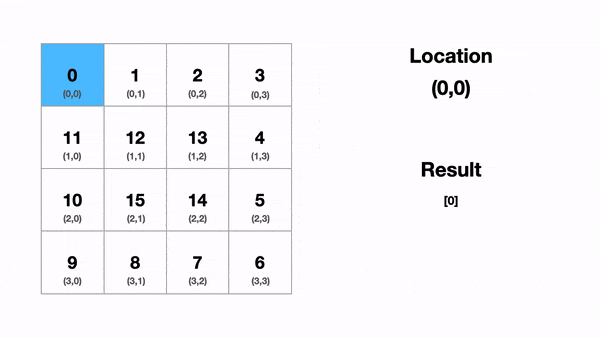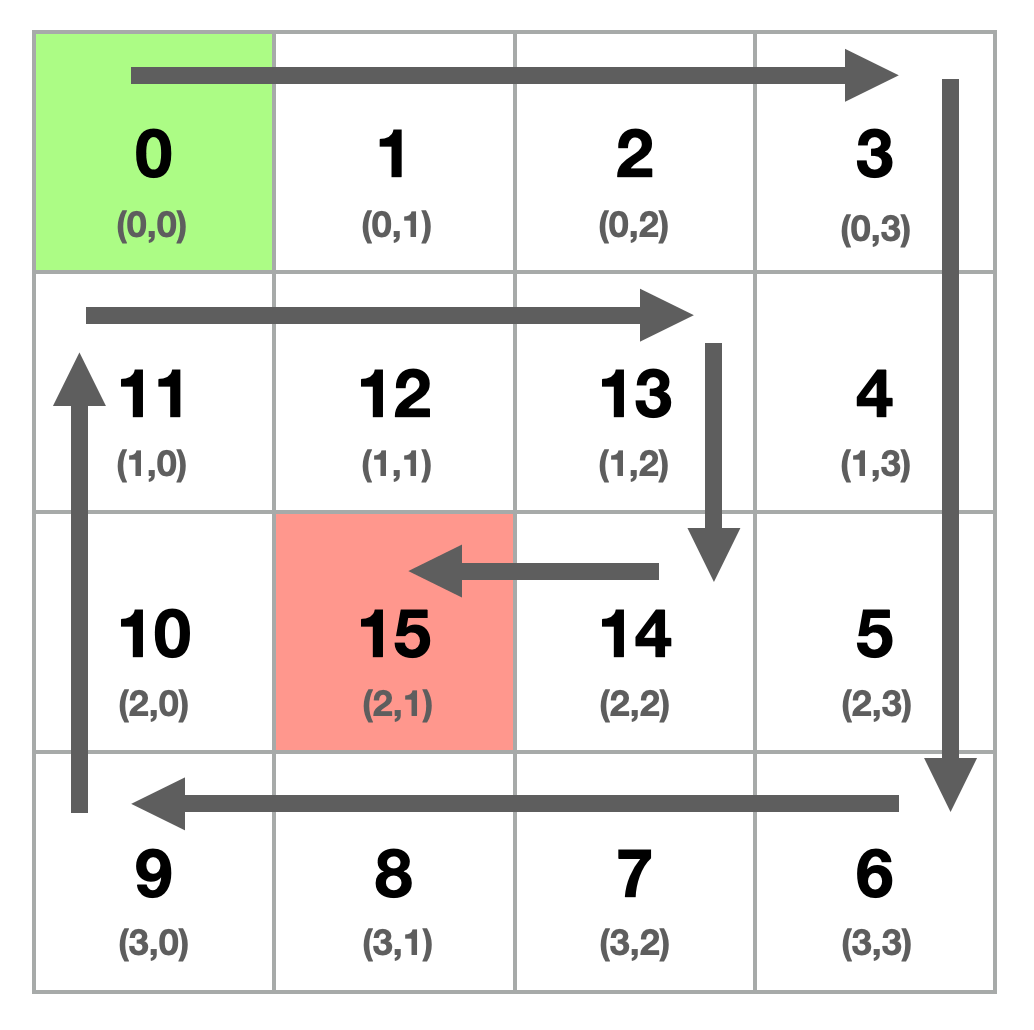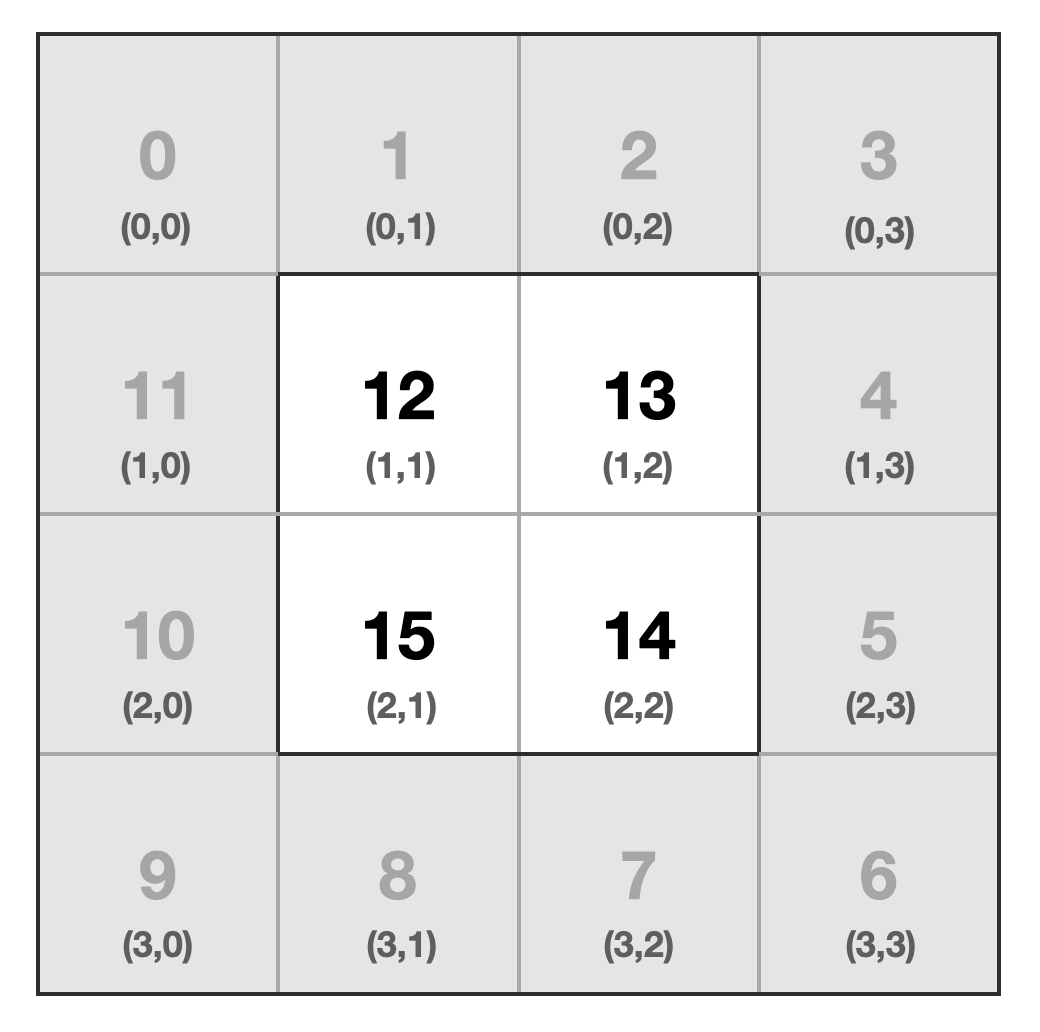Coding Interview Questions + Land Your Dev Job | Skilled.dev

# Ace Your Coding Interviews

Walk into your coding interviews feeling like you own the room.
Feel empowered to land your dream job.
Confidently answer any technical question.
Never bomb an interview again.
Skilled.dev contains years of interview expertise refined into a single platform, carefully curated to teach you exactly what you need to know to crush your coding interviews and land the job. I guide you step-by-step and provide all the tools to make it incredibly easy to prepare for interviews.
My name is Trey, and I'll be your teacher. Get to know me better on the about page and my journey as a software engineer where I received a Google offer after my first year of experience and deeply immersed in the interview process while working in Silicon Valley startups. Or keep scrolling if you want to learn more about the course.
_Upgrade Now, Study Later.

## Lock in your price by buying a gift card and activate it when you're ready to start studying to land your next job

If you're not ready to start the course now, you can still lock in the current price by buying a gift card for yourself.
Waiting for your language to be added
Not on the job hunt
Don't have time for a full course
Gift cards are automatically added to your account and can be redeemed anytime from the settings page for full access.66 articles + videos
More programming languages coming very soon_Solution Videos.

_Code Execution.

## The best way to learn anything in programming is by writing code

_Guided Articles.

## Break down how to think through each question step-by-step and solve the problem at your own pace

You are given a `matrix` represented by an `r x c` two-dimensional array of integers. Starting at the root `matrix[row = 0][column = 0]` and walking from the perimeter of the matrix towards the center, you want to touch each element once in the matrix by traversing it clockwise in spiral order. Return a 1-dimensional array of all the elements from the matrix in the order you visited them.

Write a function `walkMatrix` that takes an `r x c` 2D array and returns a 1D array of all the elements in the matrix printed in clockwise order.

Note: Do not consider the result array when calculating your space complexity.

``````// Input
const matrix = [
[0, 1, 2, 3],
[11, 12, 13, 4],
[10, 15, 14, 5],
[9, 8, 7, 6],
];

// Output: walkMatrix(matrix)
[0, 1, 2, 3, 4, 5, 6, 7, 8, 9, 10, 11, 12, 13, 14, 15];
``````

## Breakdown

Let's begin by tracing this out by hand and understanding what is happening.Or if we viewed it as paths:Let's start from the beginning. We start at the top-left (0,0) point. We walk across the first row from the first column to the last column. We are at the top-right point (0,3).

Once we reach this point, we have come to the end of the first side. Now we walk down the final column across all the rows. Once we reach the final row, we have completed traversing this side and are at the bottom-right point (3,3).

We then walk in the reverse order through the final row from the last column to the first column, reaching the bottom-left point (3,0).

To complete this first loop, we walk up the left side. However, since we have already visited (0,0), we end this loop at point (1,0).

To summarize, we walk the entire length of each side right-down-left-up for the perimeter. On the up-traversal though, we stop 1 spot back since we had already visited that point.

Now if we want to continue our traversal, we see that our problem looks identical to how we started. We need to walk the "new" perimeter and move the final index of each side inward by one.Can you take this logic and transcribe it into code?

The logic seems straight forward. We walk an entire side until we reach a node that we have already visited. Then we turn 90° and walk the next side. We repeat this pattern until we reach the center of the matrix which means there are no unvisited nodes in our path.

This now presents three questions:

1. How do we determine if we have visited a node?
2. How will we walk the matrix in code?
3. When do we know that we've reached the center of the matrix?

If your mind immediately thought of a hash table to track the visited cells, I want to applaud you 👏👏. This is usually a good first intuition and means you understand the value of constant time O(1) inserts/lookups. However, we also know that using a hash table adds an additional linear O(n) space to our solution.

Looking back at the path of our matrix walk, we see that when we reach our starting point after walking the perimeter, we ended one index back at (1,0). If we walk each side again, we can see that this happens every time — we just end one index closer to the center.

It is the same problem repeated over and over but just shifted inward. If we solve it once, we can repeat the logic for every loop of the matrix until we reach the center.

Since we walk this repeating pattern and simply shift an index towards the center each time we complete a side, we can derive the visited nodes from the position we are at during the walk with a lower and upper bounding index. We will need an index to track the start and end for both columns and rows. This means we can do it using 4 variables which reduces our space cost to constant O(1), beating our hash table.

Now let's code out how we would walk the initial perimeter so we can gain a better intuition of how the indexes work.

Taking our initial walk around the perimeter:

1. We walk the full size of the first row (beginning to end)
2. We walk the full size of the last column (beginning to end)
3. We walk the full size of the last row (end to beginning)
4. We walk the full size - 1 of the first column (end to beginning - 1)

If we translate that to code, it would look like the following:

``````// All row in the matrix
const rowCount = matrix.length;
// All columns in the 0th row
const columnCount = matrix.length;

// Walk right (top row)
for (let column = 0; column < columnCount - 1; column++) {
const nodeInFirstRow = matrix[column];
}

// Walk down (right column)
for (let row = 0; row < rowCount - 1; row++) {
const nodeInLastColumn = matrix[row][columnCount - 1];
}

// Walk left (bottom row)
for (let column = columnCount - 1; column >= 0; column--) {
const nodeInLastRow = matrix[rowCount - 1][column];
}

// Walk up (left column)
// NOTE: notice stop before the 0th node at (1,0)
for (let row = rowCount - 1; row >= 1; row--) {
const nodeInFirstColumn = matrix[row];
}
``````

As the code shows, tracking indexes and making sure you aren't off by 1 is very important for this problem.

We can see that when we walk right, we start at 0 and go to the length - 1. Then when we walk left we start at length - 1 and walk backward to 0. For down it's the same, but then when we go back up, we stop one spot early.

Then if we started our perimeter walk again, we are now initially at (1,1) and would end at (1, length - 2). This would be the same for each side. We increase our lower bounds by 1 and decrease the upper bounds by 1.

Once you complete a side, you know that it will no longer be available for access, so you can bring the bounding side in by 1 index. To generalize the code we just wrote in this manner, it would become:

``````const rowCount = matrix.length;
const columnCount = matrix.length;
let startRow = 0;
let endRow = rowCount - 1;
let startColumn = 0;
let endColumn = columnCount - 1;

// Walk right (top row)
for (let column = startColumn; column <= endColumn; column++) {
const nodeInFirstRow = matrix[startRow][column];
}
// We have walk across the entirety of the starting row, so increment the index.
startRow++;

// Walk down (right column)
for (let row = startRow; row <= endRow; row++) {
const nodeInLastColumn = matrix[row][endColumn];
}
// We have walked across end column, so we decrement the index.
endColumn--;

// Walk left (bottom row)
for (let column = endColumn; column >= startColumn; column--) {
const nodeInLastRow = matrix[endRow][column];
}
// We have walked across end row, so we decrement the index.
endRow--;

// Walk up (left column)
for (let row = endRow; row >= startRow; row--) {
const nodeInFirstColumn = matrix[row][startColumn];
}
// We have walked across the starting column, so we increment the index
startColumn++;
``````

Our indexing is much more logically tracked.

So we know how to walk a perimeter, and it is generalized to handle when we step inward and tighten up the bounds. How do we know when we have visited every node and can stop iterating?

We continue to walk the matrix inwards until we visit all the nodes. Intuitively we know that we have finished the problem when the start and end indexes collapse in on themselves, which means the start row equals the end row, and the start column equals the end column. If the bounds are equal or move past each other, all the options have been used. We will iterate through the matrix `while` the end row/column is greater than or equal to the start row/column. Translated to code: `while (endRow >= startRow && endColumn >= startColumn)`

Now let's consider an edge case. What if there is only 1 row or column remaining? If we have a final row with the numbers `[100, 200, 300]`, we want to make sure we iterate across correctly on the first pass `start -> end`, but we want to make sure that we don't iterate back across the values when we go `end -> start` since they have already been accounted for. So before we begin our last two `for` loops, we need to ensure our start/end indexes are still within the bounds since we updated the bounds after the first two loops for the rows and columns.

Alright, we're tracking visited nodes, walking the array inwards, and stopping when our indexes pass the upper and lower bounds. Let's put this all together in a working solution.

### Validate My Answer

1. You can do this in linear time O(n) and only need to visit each cell once.

2. You may be tempted to use an additional data structure which would add an additional linear O(n) space. Based on the board dimensions and location, you can actually solve this with constant space O(1).

3. Ensure you handle your indexes well and don't have off-by-one when tracking or let them bleed into cells you have already visited.

4. Does your solution handle any board dimension `r x c`? The input can be a square, vertical rectangle, or horizontal rectangle.

5. Does your solution handle an empty matrix `[[]]` `0 x 0` input?

6. Does your solution handle a `1 x c` and `r x 1` input correctly without repeating values?

_Course Outline.

## Carefully curated to contain everything you need to know in a single platform to ace your coding interviews

Big O
Arrays
Hash Tables
Strings
Recursion
Searching and Sorting
Linked Lists
Stacks and Queues
Trees
Graphs
Dynamic Programming
JavaScript
Questions Handpicked to Ace Interviews at Companies Like

## Land your dream job.

Walk into your coding interviews feeling like you own the room.